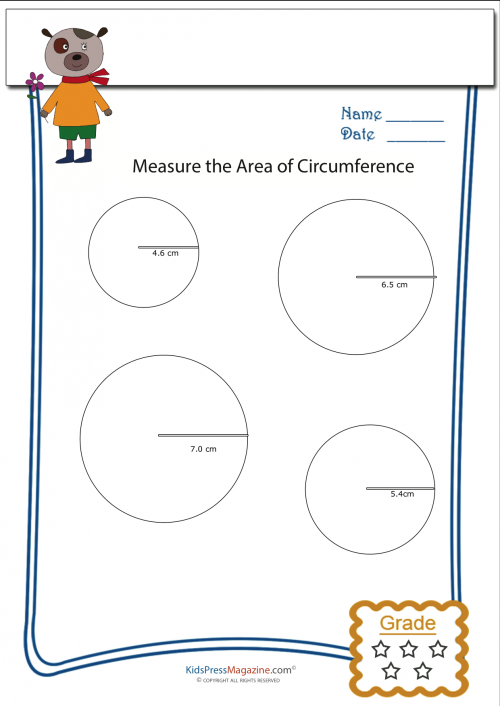# Measure Circumference Worksheet 1

The circumference of a circle is the distance around the outside of the circle. To find the circumference of a circle simply multiply the diameter of the circle by Pi (3.14). Remember – the diameter of a circle is always the radius multiplied by 2.